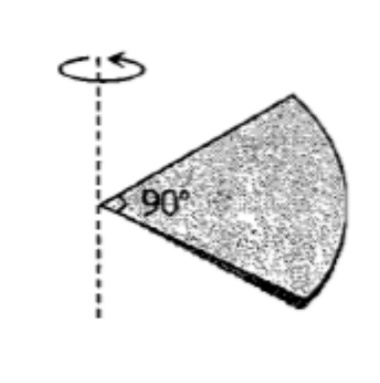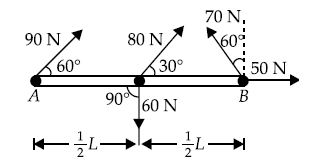A particle of mass 1 kg is kept at (1m, 1m, 1m). The moment of inertia of this particle about z-axis would be

1.

2.

3.

4.  None of these

High Yielding Test Series + Question Bank - NEET 2020

Difficulty Level:

One quarter sector is cut from a uniform circular disc of radius R.  This sector has mass M.  It is made to rotate about a line perpendicular to its plane and passing through the centre of the original disc.  Its moment of inertia about the axis of rotation is [IIT-JEE (Screening) 2001](1) $\frac{1}{2}M{R}^{2}$

(2) $\frac{1}{4}M{R}^{2}$

(3) $\frac{1}{8}M{R}^{2}$

(4) $\sqrt{2}M{R}^{2}$

High Yielding Test Series + Question Bank - NEET 2020

Difficulty Level:

A wheel is rotating at the rate of 33 rev/min.  If it comes to stop in 20 s. Then, the angular retardation will be

(1) $\mathrm{\pi }\frac{\mathrm{rad}}{{\mathrm{s}}^{2}}$                          (2)

(3)               (4)

High Yielding Test Series + Question Bank - NEET 2020

Difficulty Level:

A solid sphere is rotating about a diameter at an angular velocity $\omega$.  If it cools so that its radius reduces to $\frac{1}{n}$ of its  original value, its angular velocity becomes [MP PMT 2006]

(1) $\frac{\omega }{n}$                                (2) $\frac{\omega }{{n}^{2}}$

(3) $n\omega$                                (4) ${n}^{2}\omega$

High Yielding Test Series + Question Bank - NEET 2020

Difficulty Level:

A horizontal platform is rotating with uniform angular velocity around the vertical axis passing through its centre. At some instant of time a viscous fluid of mass 'm' is dropped at the centre and is allowed to spread out and finally fall. The angular velocity during this period

1. Decreases continuously

2. Decreases initially and increases again

3. Remains unaltered

4. Increases continuously

Concept Questions :-

Angular momentum
High Yielding Test Series + Question Bank - NEET 2020

Difficulty Level:

The total torque about pivot A provided by the forces shown in the figure, for L = 3.0 m, is :1. 210 Nm

2. 140 Nm

3. 95 Nm

4. 75 Nm

Concept Questions :-

Torque
High Yielding Test Series + Question Bank - NEET 2020

Difficulty Level:

For the same total mass, which of the following will have the largest moment of inertia about an axis passing through the centre of gravity and perpendicular to the plane of the body?

1. A disc of radius a

2. a ring of radius a

3. a square lamina of side 2 a

4. four rods forming square of side 2 a.

Concept Questions :-

Moment of inertia
High Yielding Test Series + Question Bank - NEET 2020

Difficulty Level:

The radius of gyration of a uniform rod of length L about an axis passing through its centre of mass is

1. $\frac{L}{2\sqrt{3}}$

2. $\frac{{L}^{2}}{12}$

3. $\frac{L}{\sqrt{3}}$

4. $\frac{L}{\sqrt{2}}$

Concept Questions :-

Moment of inertia
High Yielding Test Series + Question Bank - NEET 2020

Difficulty Level:

Two uniform, thin identical rods each of mass M and length l are joined together to form a cross. What will be the moment of inertial of the cross about an axis passing through the point at which the two rods are joined and perpendicular to the plane of the cross

1. $\frac{M{l}^{2}}{12}$

2. $\frac{M{l}^{2}}{6}$

3. $\frac{M{l}^{2}}{4}$

4. $\frac{M{l}^{2}}{3}$

Concept Questions :-

Moment of inertia
High Yielding Test Series + Question Bank - NEET 2020

Difficulty Level:

If the linear momentum is increased by 50%, the kinetic energy will increase by

1.  50%

2.  100%

3.  125%

4.  25%

Concept Questions :-

Linear momentum